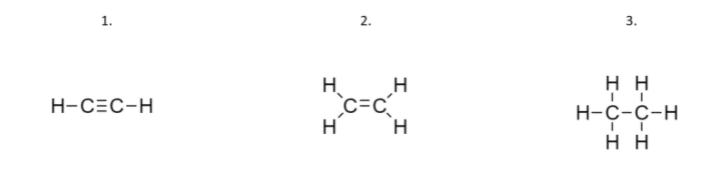# Order These Compounds In Order Of Increasing Carbon-carbon Bond Strength.

We thoroughly check each answer to a question to provide you with the most correct answers. Found a mistake? Let us know about it through the REPORT button at the bottom of the page.

Order these compounds in order of increasing carbon-carbon bond strength and in order of decreasing carbon-carbon bond length: HCCH, H2CCH2, H3CCH3.

Contents

We need to list these compounds in order of increasing carbon-carbon bond strength and ion order to decrease the carbon-carbon bond length.

Let us first draw the Lewis structures of the compounds:

1. compound: Here we have only 2 hydrogen atoms, so we need to draw a triple bond between carbon atoms.

2. compound: Here we have 4 hydrogen atoms, so we need to draw a double bond between carbon atoms.

3. compound: Here we have 6 hydrogen atoms, so we have a single bond between carbon atoms.Increasing bond strength: we need to start with the weakest carbon bond. It will be the single bond, the stronger bond is the double bond, and the strongest one is the triple bond. The more bonds we have, the stronger the bond is.

Therefore, the increasing bond strength list is: 3. compound, 2. compound,1. compound.

Decreasing bond length: we need to start with the longest bond length. Stronger bonds pull the atoms closer together. So, the longest bond will be the single bond, then the double bond, and the shortest will be the triple bond.

Therefore, the decreasing bond length list is: 3. compound, 2. compound, 1. compound.

We can see that there is the same trend in both lists: 3. compound, 2. compound, 1. compound.

## Result

The increasing bond strength and the decreasing bond length list: 3. compound, 2. compound, 1. compound.